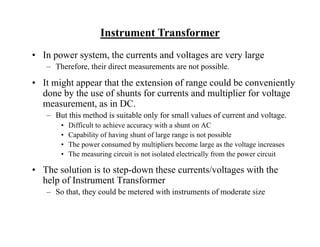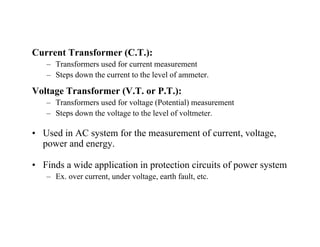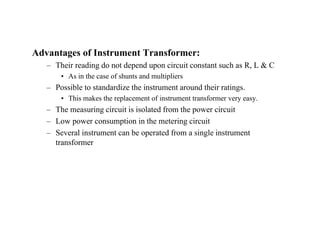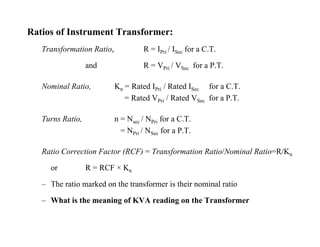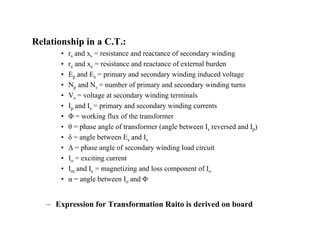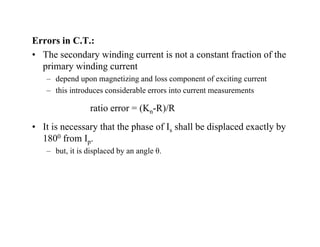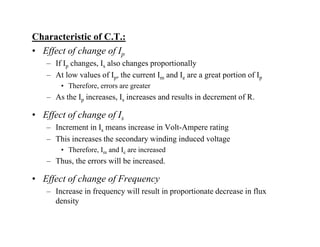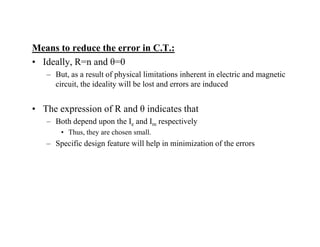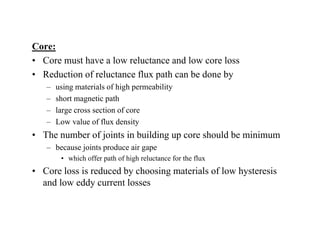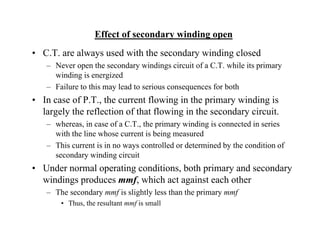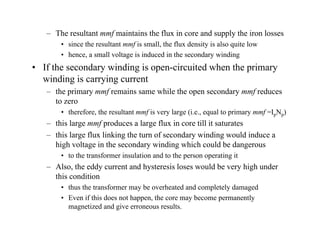1 of 12

### InstrumentTransformer.pdf

1. Instrument Transformer • In power system, the currents and voltages are very large – Therefore, their direct measurements are not possible. • It might appear that the extension of range could be conveniently done by the use of shunts for currents and multiplier for voltage measurement, as in DC. , – But this method is suitable only for small values of current and voltage. • Difficult to achieve accuracy with a shunt on AC • Capability of having shunt of large range is not possible • The power consumed by multipliers become large as the voltage increases • The measuring circuit is not isolated electrically from the power circuit • The solution is to step down these currents/voltages with the • The solution is to step-down these currents/voltages with the help of Instrument Transformer – So that, they could be metered with instruments of moderate size
2. Current Transformer (C.T.): – Transformers used for current measurement – Steps down the current to the level of ammeter. Steps down the current to the level of ammeter. Voltage Transformer (V.T. or P.T.): – Transformers used for voltage (Potential) measurement – Steps down the voltage to the level of voltmeter. • Used in AC system for the measurement of current, voltage, power and energy. • Finds a wide application in protection circuits of power system – Ex. over current, under voltage, earth fault, etc.
3. Advantages of Instrument Transformer: – Their reading do not depend upon circuit constant such as R, L & C • As in the case of shunts and multipliers • As in the case of shunts and multipliers – Possible to standardize the instrument around their ratings. • This makes the replacement of instrument transformer very easy. Th i i it i i l t d f th i it – The measuring circuit is isolated from the power circuit – Low power consumption in the metering circuit – Several instrument can be operated from a single instrument transformer
4. Ratios of Instrument Transformer: Transformation Ratio, R = IPri / ISec for a C.T. and R = VPri / VSec for a P.T. Nominal Ratio, Kn = Rated IPri / Rated ISec for a C.T. = Rated VPri / Rated VSec for a P.T. Turns Ratio, n = Nsec / NPri for a C.T. = NPri / NSec for a P.T. Ratio Correction Factor (RCF) = Transformation Ratio/Nominal Ratio=R/Kn or R = RCF × Kn or R RCF Kn – The ratio marked on the transformer is their nominal ratio – What is the meaning of KVA reading on the Transformer What is the meaning of KVA reading on the Transformer
5. C t T f Current Transformer: – Primary winding is connected in series with line carrying the current to be measured • Therefore, IPri α Load – Primary winding consist of very few turns • Therefore no appreciable voltage drop across it • Therefore, no appreciable voltage drop across it – Secondary winding has larger number of turns • Exact number is being determined by the turn ratio
6. Relationship in a C.T.: p • rs and xs = resistance and reactance of secondary winding • re and xe = resistance and reactance of external burden • Ep and Es = primary and secondary winding induced voltage p d s p y d seco d y w d g duced vo ge • Np and Ns = number of primary and secondary winding turns • Vs = voltage at secondary winding terminals • Ip and Is = primary and secondary winding currents Ip and Is primary and secondary winding currents • Φ = working flux of the transformer • θ = phase angle of transformer (angle between Is reversed and Ip) • δ = angle between Es and Is δ angle between Es and Is • Δ = phase angle of secondary winding load circuit • Io = exciting current • I and I = magnetizing and loss component of I Im and Ie magnetizing and loss component of Io • α = angle between Io and Φ i f f i i i i – Expression for Transformation Raito is derived on board
7. Errors in C.T.: • The secondary winding current is not a constant fraction of the primary winding current d d ti i d l t f iti t – depend upon magnetizing and loss component of exciting current – this introduces considerable errors into current measurements ratio error = (K R)/R ratio error = (Kn-R)/R • It is necessary that the phase of Is shall be displaced exactly by 1800 from I 180 from Ip. – but, it is displaced by an angle θ.
8. Characteristic of C.T.: • Effect of change of Ip – If Ip changes, Is also changes proportionally – At low values of I , the current I and I are a great portion of I At low values of Ip, the current Im and Ie are a great portion of Ip • Therefore, errors are greater – As the Ip increases, Is increases and results in decrement of R. • Effect of change of Is – Increment in Is means increase in Volt-Ampere rating This increases the secondary winding induced voltage – This increases the secondary winding induced voltage • Therefore, Im and Ie are increased – Thus, the errors will be increased. • Effect of change of Frequency – Increase in frequency will result in proportionate decrease in flux density density
9. M t d th i C T Means to reduce the error in C.T.: • Ideally, R=n and θ=0 – But, as a result of physical limitations inherent in electric and magnetic But, as a result of physical limitations inherent in electric and magnetic circuit, the ideality will be lost and errors are induced • The expression of R and θ indicates that • The expression of R and θ indicates that – Both depend upon the Ie and Im respectively • Thus, they are chosen small. – Specific design feature will help in minimization of the errors
10. Core: Core: • Core must have a low reluctance and low core loss • Reduction of reluctance flux path can be done by Reduction of reluctance flux path can be done by – using materials of high permeability – short magnetic path l i f – large cross section of core – Low value of flux density • The number of joints in building up core should be minimum j g p – because joints produce air gape • which offer path of high reluctance for the flux • Core loss is reduced by choosing materials of low hysteresis • Core loss is reduced by choosing materials of low hysteresis and low eddy current losses
11. Effect of secondary winding open • C.T. are always used with the secondary winding closed – Never open the secondary windings circuit of a C.T. while its primary winding is energized winding is energized – Failure to this may lead to serious consequences for both • In case of P.T., the current flowing in the primary winding is l l h fl i f h fl i i h d i i largely the reflection of that flowing in the secondary circuit. – whereas, in case of a C.T., the primary winding is connected in series with the line whose current is being measured – This current is in no ways controlled or determined by the condition of secondary winding circuit • Under normal operating conditions both primary and secondary Under normal operating conditions, both primary and secondary windings produces mmf, which act against each other – The secondary mmf is slightly less than the primary mmf Th th lt t f i ll • Thus, the resultant mmf is small
12. – The resultant mmf maintains the flux in core and supply the iron losses • since the resultant mmf is small the flux density is also quite low since the resultant mmf is small, the flux density is also quite low • hence, a small voltage is induced in the secondary winding • If the secondary winding is open-circuited when the primary i di i i t winding is carrying current – the primary mmf remains same while the open secondary mmf reduces to zero • therefore, the resultant mmf is very large (i.e., equal to primary mmf =IpNp) – this large mmf produces a large flux in core till it saturates – this large flux linking the turn of secondary winding would induce a g g y g high voltage in the secondary winding which could be dangerous • to the transformer insulation and to the person operating it – Also, the eddy current and hysteresis loses would be very high under , y y y g this condition • thus the transformer may be overheated and completely damaged • Even if this does not happen, the core may become permanently pp y p y magnetized and give erroneous results.